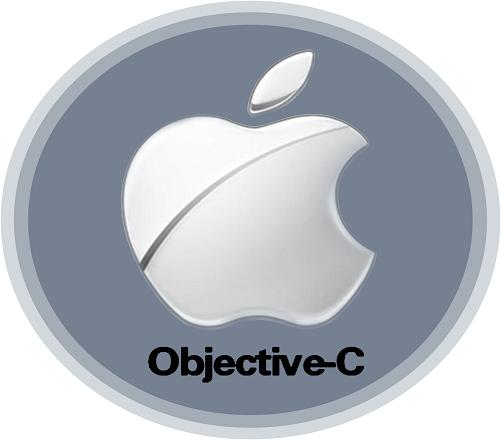# How Much Do You Know Objective-c Coding Test?

10 Questions | Total Attempts: 120SettingsAs one of the programming languages that were derived from the C programming language, Objective-C has been and is being used by many companies to build their apps. Apple used it to build their OS X and iOS operating systems. Go ahead and take the quiz to get an idea of how much you know of this programming language.

Related Topics
• 1.
What will a class definition NOT contain?
• A.

An interface section

• B.

A main routine

• C.

Instance variables

• D.

Methods

• 2.
What is a local variable that has no default initial value and does not retain its value through method calls?
• A.

A static variable

• B.

An auto variable

• C.

A global variable

• D.

None of the above

• 3.
What is the value of sum after executing this loop: for (int i = 0, sum = 0;      i < 10; i += 2)    sum += i;
• A.

30

• B.

20

• C.

17

• D.

55

• 4.
What does Data Encapsulation mean?
• A.

Putting some information in a time capsule and burying it in your backyard

• B.

Storing an object's data in its instance variables with access through its methods

• C.

Putting all the data inside the program code

• D.

Archiving data to a file so it can later be read back in

• 5.
What is the value of sum after executing this loop: for (int i = 0, sum = 0;      i < 15; i += 2)    sum += i;
• A.

20

• B.

55

• C.

30

• D.

17

• 6.
What is the difference between #import and #include
• A.

The former prevents a file from being included multiple times

• B.

The latter is recommended for use by Apple

• C.

The former checks the current directory as well as the system directories

• D.

There is no difference

• 7.
What is true about the local variable counter if this statement is executed inside a method? static int counter = 0;
• A.

Its value will be set to 0 only once when the program begins execution

• B.

Its value will not be retained through method calls

• C.

Its value will be set to 0 every time the method is called

• D.

None of the above

• 8.
Given i = 15, what is the value of this expression?: i / 2.0
• A.

The floating value 3.141592654

• B.

The floating value 7.0

• C.

The integer value 7

• D.

The floating value 7.5

• 9.
If x and y are integers, what will the statement do? if ( x = 5 )     y = 0; else    y = 1;
• A.

Will assign 1 to y

• B.

Will assign 0 to y

• C.

Will assign either 0 or 1 to y based on the value of x

• D.

Is not a valid statement

• 10.
A deallocmethod should:
• A.

Release its instance variables only

• B.

Release its instance variables, then send a dealloc message to super

• C.

First invoke dealloc on super , then release its instance variables

• D.

None of the above